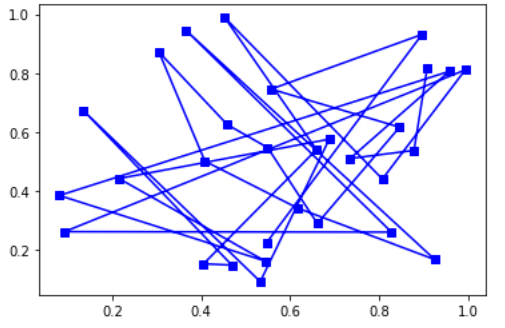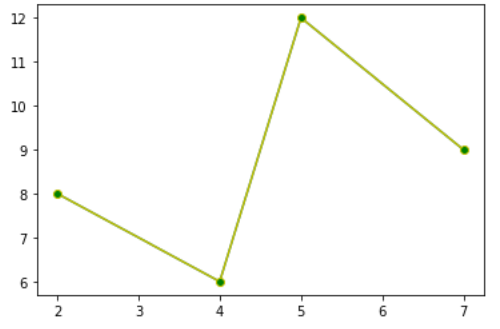# matplotlib.lines.VertexSelector class in Python

The matplotlib.lines.VertexSelector class belongs to the matplotlib.lines module. The matplotlib.lines module contains all the 2D line class that can be drawn with a variety of line styles, markers and colors.` matplotlib.lines.VertexSelector` class is used to for managing the callbacks that maintain a list of selected vertices for matplotlib.lines.Line2D. The `process_selected()` function gets overridden by the derived classes for the picks.

Syntax: class matplotlib.lines.VertexSelector(line)

Parameter:

• lines: These represent the lines that are defined for the plotting.

Methods of the class:

1. onpick(self, event): The selected set of indices gets updated when the line is picked using this method.
2. process_selected(self, ind, xs, ys): By default it does the implementation of “do nothing” of the process_selected() method. The ‘ind’ represents the indices of the selected vetrices whereas ‘xs’ and ‘ys’ are the coordinates of the selected vertices.

Example #1:

 `import` `numpy as np ` `import` `matplotlib.pyplot as plt ` `import` `matplotlib.lines as lines ` ` `  ` `  `# class for highlighting selected area ` `class` `Highlighter(lines.VertexSelector): ` `     `  `    ``# constructor for the highlighter class ` `    ``def` `__init__(``self``, line, fmt ``=``'ro'``, ``*``*``kwargs): ` `        ``lines.VertexSelector.__init__(``self``, line) ` `        ``self``.markers, ``=` `self``.axes.plot([], [], fmt, ``*``*``kwargs) ` `     `  `    ``# helper function process selected area and plot graph accordingly ` `    ``def` `process_selected(``self``, ind, xs, ys): ` `        ``self``.markers.set_data(xs, ys) ` `        ``self``.canvas.draw() ` ` `  `figure, ax ``=` `plt.subplots() ` `x_axis, y_axis ``=` `np.random.rand(``2``, ``30``) ` `line, ``=` `ax.plot(x_axis, y_axis, ``'bs-'``, picker ``=` `5``) ` ` `  `selector ``=` `Highlighter(line) ` `plt.show() `

Output:Example 2:

 `import` `matplotlib.pyplot as plt ` `import` `matplotlib.lines as lines ` `from` `matplotlib.collections ``import` `PathCollection ` ` `  `lines.VertexSelector ` ` `  `# class for plot clicked area ` `class` `dragged_lines: ` `     `  `     ``# constructor for the dragged_lines class ` `    ``def` `__init__(``self``, ax): ` `        ``self``.ax ``=` `ax ` `        ``self``.c ``=` `ax.get_figure().canvas ` ` `  `        ``self``.line ``=` `lines.Line2D(x, y, picker ``=` `5``, marker ``=``'o'``, ` `                              ``markerfacecolor ``=``'g'``, color ``=``'y'``) ` ` `  `        ``self``.ax.add_line(``self``.line) ` `        ``self``.c.draw_idle() ` `        ``self``.sid ``=` `self``.c.mpl_connect(``'pick_event'``, ``self``.lineclicker) ` ` `  `    ``def` `lineclicker(``self``, event): ` `        ``if` `event.artist: ` `            ``print``(``"line selected "``, event.artist) ` `            ``self``.follower ``=` `self``.c.mpl_connect(``"motion_notify_event"``, ` `                                                 ``self``.mouse_follower) ` `            ``self``.releaser ``=` `self``.c.mpl_connect(``"button_press_event"``,  ` `                                            ``self``.release_after_click) ` ` `  `    ``def` `mouse_follower(``self``, event): ` `        ``self``.line.set_data([event.xdata, event.ydata]) ` `        ``self``.c.draw_idle() ` ` `  `    ``def` `release_after_click(``self``, event): ` `        ``data ``=` `self``.line.get_data() ` `        ``print``(data) ` ` `  `        ``self``.c.mpl_disconnect(``self``.releaser) ` `        ``self``.c.mpl_disconnect(``self``.follower) ` ` `  `figure ``=` `plt.figure() ` `axes ``=` `figure.add_subplot(``111``) ` `x_axis, y_axis ``=` `[``2``, ``4``, ``5``, ``7``], [``8``, ``6``, ``12``, ``9``] ` `axes.plot(x_axis, y_axis) ` `Vline ``=` `dragged_lines(axes) ` `plt.show() `

Output:My Personal Notes arrow_drop_upCheck out this Author's contributed articles.

If you like GeeksforGeeks and would like to contribute, you can also write an article using contribute.geeksforgeeks.org or mail your article to contribute@geeksforgeeks.org. See your article appearing on the GeeksforGeeks main page and help other Geeks.

Please Improve this article if you find anything incorrect by clicking on the "Improve Article" button below.

Article Tags :

Be the First to upvote.

Please write to us at contribute@geeksforgeeks.org to report any issue with the above content.